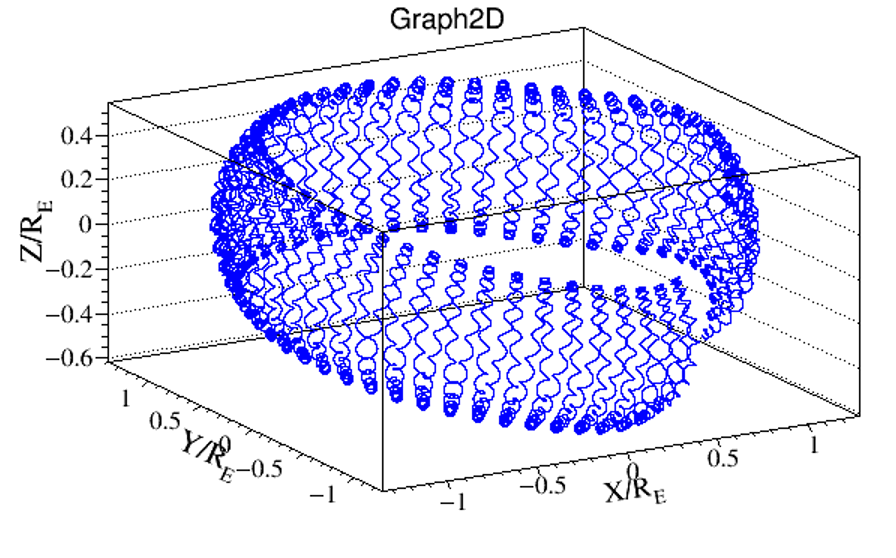When galactic charged cosmic rays reached our Solar System, their propagation is influenced by the activity of the Sun. Inside this region, called heliosphere, the interactions between particles and the small scale irregularities of the magnetic field are described by a diffusion process (see HelMod webpage).

Cosmic rays approaching our planet interact with the Earth magnetic field (composed by two contributions: an internal magnetic field and external magnetic field) that changes their directions. The geomagnetic field is produced by currents flowing inside the Earth (probably due to the Earth nucleus) and currents due to charge motion outside the planet. This field can be represented, as first approximation, by a dipole with an axis tilted of $$\sim11^{\circ}$$ respect to the Earth rotational axis and shifted with respect to it; its orientation is also opposite to the rotational axis. The region affected by this field is called magnetosphere, which extends from about 6 to 12 Earth radii (the average Earth radius is $$R_E\sim6371$$ km) in the Sun direction. This limit varies with respect to the magnetic field carried out from the Sun by the solar wind. On the opposite side of the Sun, the magnetosphere extends like the tail of a comet up to about a thousand Earth radii. Cosmic rays move following the trajectory’s of charged particles inside a magnetic field. For low energy particles the motion is divided into three components: a spiral motion around the field lines, a North-South motion along these lines and a drift motion East-West.

The effects of the geomagnetic field, on charged particles approaching the Earth, are mainly two. First of all, the trajectory of the incoming particle is curved leading to an arrival direction different from the one without the magnetic field. Second, an energy selection excludes low energy cosmic rays to reach the surface. The minimum rigidity value of a particle to enter from the outer magnetosphere is known as geomagnetic rigidity cut-off ($$R_{cut}$$). It depends on the arrival direction of the particles and the position of the observer located in the vicinity of the Earth surface. Particles with rigidity lower than the cut-off, which are located inside the magnetosphere, are trapped, and in particular climatic conditions and magnetic properties, they can create the polar aurorae; but if they are outside the magnetosphere, they can not penetrate inside and are reflected toward the interstellar space. Störmer (1955) described the geomagnetic rigidity cut-off as follow:
\begin{equation}
R_{cut}=\frac{M}{r^{2}}\frac{\cos^{4}\Lambda}{\left(\sqrt{1-\sin\xi\sin\varphi\cos^{3}\Lambda}+1\right)^{2}},
\end{equation}
where $$M$$ is the strength of the dipole moment, $$r$$ the distance from the dipole centre, $$\xi$$ the angle between the arrival direction with respect to the local magnetic Zenith, $$\Lambda$$ the geomagnetic latitude and $$\varphi$$ the arrival azimuthal angle of a positive particle. This last angle is null if the particles come from West, 90$$^{\circ}$$ if they come perpendicular on the surface and 180$$^{\circ}$$ if they come from East. Particles perpendicular to the surface, with same rigidity, prefer enter to the pole instead of the equator, in fact, the cut-off is proportional to $$(\cos^{4}\Lambda)$$. The maximum cut-off on the surface (with $$\varphi=180^{\circ}$$) is $$R_{cut}=M/R_{E}^{2}\simeq59.6\:$$GV. Under cut-off only secondary particles, produced by interactions of primary cosmic rays with the atoms of the atmosphere and trapped by the geomagnetic field, are observable. Störmer's rigidity cut-off decreases if the detection geomagnetic latitude increases and also if particles arrival direction moves westward (East-West effect). Finally, the cosmic ray intensity decreases increasing the rigidity cut-off. Thus, we expect an increment of the intensity if we move our observation direction from East to West and detection zone from equatorial to polar regions.

A good description of the geomagnetic field is needed for untangling primary cosmic rays (coming from the outer magnetosphere) from secondary cosmic rays produced in the atmosphere or trapped in the radiation belts. The rigidity value used for discriminating these two families is the geomagnetic rigidity cut-off. The Störmer cut-off (Eq. 1) is not time dependent, while the magnetic field is variable (secular variation of the dipole orientation and intensity) and depending also from the solar activity. Another way to distinguish between the two particle's families is to do the so called back-tracing, in other words, to reconstruct the particle trajectory back in time from the detection position up to the magnetosphere border.Trajectory of a secondary cosmic proton evaluated with the GeoMagSphere code